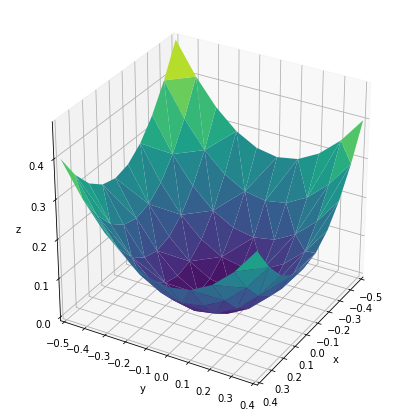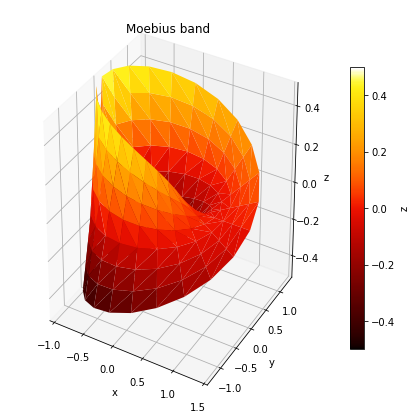# Trisurface¶

Title
TriSurface Element
Dependencies
Matplotlib
Backends
Matplotlib
In :
import numpy as np
import holoviews as hv
hv.extension('matplotlib')The TriSurface Element renders any collection of 3D points as a surface by applying Delaunay triangulation. It is therefore useful for plotting an arbitrary collection of datapoints as a 3D surface. Like other 3D elements it supports azimuth, elevation and distance plot options to control the camera position:

In :
y,x = np.mgrid[-5:5, -5:5] * 0.1
heights = np.sin(x**2+y**2)
hv.TriSurface((x.flat,y.flat,heights.flat)).opts(
azimuth=30, elevation=30, fig_size=200)

Out:Like all other colormapped plots we can easily add a colorbar and control the cmap of the plot:

In :
u=np.linspace(0,2*np.pi, 24)
v=np.linspace(-1,1, 8)
u,v=np.meshgrid(u,v)
u=u.flatten()
v=v.flatten()

#evaluate the parameterization at the flattened u and v
tp=1+0.5*v*np.cos(u/2.)
x=tp*np.cos(u)
y=tp*np.sin(u)
z=0.5*v*np.sin(u/2.)

surface = hv.TriSurface((x, y, z), label='Moebius band')
surface.opts(cmap='fire', colorbar=True, fig_size=200)

Out:For full documentation and the available style and plot options, use hv.help(hv.TriSurface).